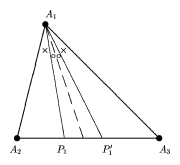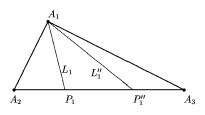# Isogonal

Literally "same angle" . There are several concepts in mathematics involving isogonality.

## Contents

### Isogonal trajectory.

A trajectory that meets a given family of curves at a constant angle. See Isogonal trajectory.

### Isogonal mapping.

A (differentiable) mapping that preserves angles. For instance, the stereographic projection of cartography has this property [a2]. See also Conformal mapping; Anti-conformal mapping.

### Isogonal circles.

A circle is said to be isogonal with respect to two other circles if it makes the same angle with these two, [a1].

### Isogonal line.

Given a triangle $A _ { 1 } A _ { 2 } A _ { 3 }$ and a line $L_1$ from one of the vertices, say from $A _ { 1 }$, to the opposite side. The corresponding isogonal line $L _ { 1 } ^ { \prime }$ is obtained by reflecting $L_1$ with respect to the bisectrix in $A _ { 1 }$.

If the lines $L _ { 1 } = A _ { 1 } P _ { 1 }$, $L _ { 2 } = A _ { 2 } P _ { 2 }$ and $L _ { 3 } = A _ { 3 } P _ { 3 }$ are concurrent (i.e. pass through a single point $X$, i.e. are Cevian lines), then so are the isogonal lines $L _ { 1 } ^ { \prime }$, $L _ { 2 } ^ { \prime }$, $L _ { 3 } ^ { \prime }$. This follows fairly directly from the Ceva theorem. The point $X ^ { \prime } = L _ { 1 } ^ { \prime } \cap L _ { 2 } ^ { \prime } = L _ { 2 } ^ { \prime } \cap L _ { 3 } ^ { \prime } = L _ { 1 } ^ { \prime } \cap L _ { 3 } ^ { \prime }$ is called the isogonal conjugate point. If the barycentric coordinates of $X$ (often called trilinear coordinates in this setting) are $( \alpha : \beta : \gamma )$, then those of $X ^ { \prime }$ are $( \alpha ^ { - 1 } : \beta ^ { - 1 } : \gamma ^ { - 1 } )$Figure: i130080a

Another notion in rather the same spirit is that of the isotomic line to $L_1$, which is the line $L_1 ^ { \prime \prime } = A _ { 2 } P ^ { \prime \prime }_1$ such that $| A _ { 2 } P _ { 1 } ^ { \prime \prime } | = | P _ { 1 } A _ { 3 } |$. Again it is true that if $L_1$, $L_{2}$, $L_3$ are concurrent, then so are $L _ { 1 } ^ { \prime \prime }$, $L _ { 2 } ^ { \prime \prime }$, $L _ { 3 } ^ { \prime \prime }$. This follows directly from the Ceva theorem.Figure: i130080b

The point $X ^ { \prime \prime } = L _ { 1 } ^ { \prime \prime } \cap L _ { 2 } ^ { \prime \prime } = L _ { 2 } ^ { \prime \prime } \cap L _ { 3 } ^ { \prime \prime } = L _ { 1 } ^ { \prime \prime } \cap L _ { 3 } ^ { \prime \prime }$ is called the isotomic conjugate point. The barycentric coordinates of $X ^ { \prime \prime }$ are $( a ^ { 2 } \alpha ^ { - 1 } : b ^ { 2 } \beta ^ { - 1 } : c ^ { 2 } \gamma ^ { - 1 } )$, where $a$, $b$, $c$ are the lengths of the sides of the triangle. The Gergonne point is the isotomic conjugate of the Nagel point.

The involutions $X \mapsto X ^ { \prime }$ and $X \mapsto X ^ { \prime \prime }$, i.e. isogonal conjugation and isotomic conjugation, are better regarded as involutions of the projective plane $\mathbf{P} ^ { 2 } ( \mathbf{R} )$, [a4].

How to Cite This Entry:
Isogonal. Encyclopedia of Mathematics. URL: http://encyclopediaofmath.org/index.php?title=Isogonal&oldid=53564
This article was adapted from an original article by M. Hazewinkel (originator), which appeared in Encyclopedia of Mathematics - ISBN 1402006098. See original article